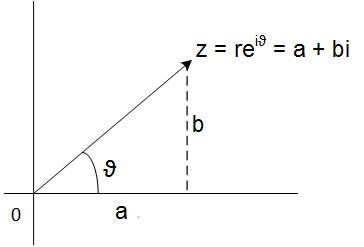# Complex numbers

### Basic concepts

Effectively, the equation $$x^{2} + 1 = 0$$ Has solution, in fact it has two solutions wich are $x = \pm \sqrt{-1} = \pm i$. Positive solution from them is called as imaginary unit.

In general, numbers on the form $$z=a+bi$$ Are called complex numbers, the a is called real part of b and b is the imaginary part of z. They are denoted respectively for $Re z=a, Im z=b$.

The complex plane consists of all complex numbers, that is, pairs of real numbers (a, b), formally $$\mathbb{C} = \{ z = a+bi : a, b \in \mathbb{R} \}$$ This notation, used for the first time by Euler in 1773, gives way to a precious and prosperous branch of analysis called Complex Variable analysis.

If we change the sign to the imaginary part we have the conjugate complex . Ie, the number $$\bar{z}=a-bi$$ It is called conjugate complex .

Note that an attentive student could claim that $\mathbb{C} = \mathbb{R} ^2$, which is true from a topological point of view, but not from an algebraic point of view because in the plane$\mathbb{C}$ there are addition and multiplication operations which do not exist a priori in the usual plane of $\mathbb{R} ^2$. Formally:

### Complex plane definition

Complex plane is defined as $$\mathbb{C} = \{ z = a+bi : a, b \in \mathbb{R} \}$$ and if $z, w \in \mathbb{C} : z = a+bi, w = c + di$ then the addition and multiplication operations respectively are defined as: $$z+w = a+c +(b+d)i$$ $$zw = (ac - bc) +(bc+ad)i$$ The complex plane endowed with these two operations has a Field structure.

### Extended theory

Every complex number can be written in the polar form , so

$z = a+bi = re^{i\theta}$

by polar form of a complex number we haveFigura 1: Polar form of complex number.

$a=r \,cos\,\theta,\: b =r \,sin\,\theta$

being r modulus of z. It is calculated as

$|z| = \sqrt{x^{2}+y^{2}}$

The argument of a complex number is well defined except multiples of $2\pi$, ie $$arg\, z = \theta + 2k\pi,\,\, k=1, 2, 3 ...$$ or $$arg\,z = \theta ,\,\,\,\ mod\,2\pi$$ We will refer, from now on, to $Arg z = \theta$, then $$arg\,z = Arg\,z + 2k\pi,\,\, k=1, 2, 3 ...$$ Suppose we want to calculate the square of z, that is, $z^{2}$, then: $$z = re^{i \theta} \Rightarrow z^{2} = r^2 e^{2i \theta} \Rightarrow arg z^{2} = 2 Arg\,z + 4k\pi,\,\, k=1, 2, 3 ...$$ When we do the inverse operation, that is, the square root, it happens that $$arg \, z^{\frac{1}{2}} = \frac{arg \, z }{2} + \frac{2k\pi}{2}$$ So, now we can calculate square roots of negative numbers, for example, to calculate the square root of -1 $$arg \, (-1)^{\frac{1}{2}} = \frac{\pi}{2} + k\pi$$. because this i and -i are both square roots of -1.

### Complex numbers operations

Given two complex numbers

$z_{1} = r_{1} e^{i \theta_{1} }$
$z_{2} = r_{2} e^{i \theta_{2} }$

It is easy

$arg\, z_{1}z_{2} = arg\, z_{1} + arg\,z_{2}$

$arg\, \frac{z_{1}}{z_{2}} = arg\, z_{1} - arg\,z_{2}$

this is because

$z_{1}z_{2} = r_{1} e^{i \theta_{1} } . r_{2} e^{i \theta_{2} } = r_{1} r_{2} e^{i (\theta_{1}+ \theta_{2}) }$

Lets use this link to see how to calculate power of complex numbers.

## Was useful? want add anything?

Post here

### Post from other users

Post here